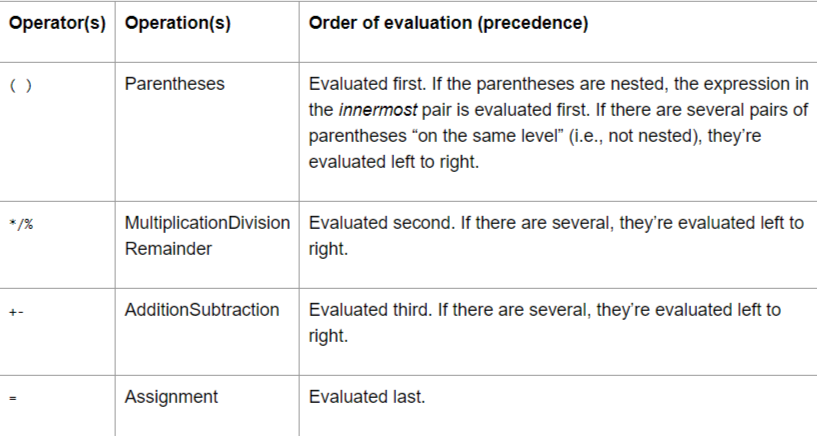ADVERTISEMENT

ADVERTISEMENT

ADVERTISEMENT

# Precedence and associativity of Arithmetic Operators in C language

In this article, we will learn about the Precedence and associativity of Arithmetic Operators in C language.
Submitted by Abhishek Pathak, on October 24, 2017

While, writing programs in C, we mostly perform calculations and arithmetic operations using the C arithmetic operators. Even the basic addition program in C involves the use of arithmetic addition operator `+` to perform addition of two integers or float value.

For example, the asterisk * indicates multiplication and the forward slash (/) denotes the division operator. Unlike algebra, in C, to multiply a times b, the multiplication must be explicitly denoted by using the * operator, as in a * b. The arithmetic operators are all binary operators. For example, the expression 3 + 7 contains the binary operator + and the operands 3 and 7, or - operator subtracts the second operand from the first.

C also has special % modulus operator, which returns the remainder of two numbers. It can be used cleverly in programs such as finding a number whether it is even or odd.

But, when operators are used in a composite expression, then the values might not be what you expect. Consider this example,

`a = 10 + 2 * 5 - 6 / 2`

If you think its 27, then you are tricked by C. All mathematical operations form a hierarchy based on priority which is shown below. In the above calculation the multiplication and division parts will be evaluated first and then the addition and subtraction parts. This gives an answer of 17.According to this table, from the left to right, the operator with higher precedence will get evaluated first. If two operators have same precedence, then the one which occurs first from left to right will get evaluated first. The parentheses have highest priority, which means expressions inside them are evaluated first. Parentheses can contain sub parentheses and the rule follows same for ever operator.

Here are a few examples to help you better,

```a = 16 + (2 * 5) - (6 / 2) // Answer: 23
a = (10 + 2) * (8 - 6) / 2 // Answer: 12
a = 15 + (4 * 12 / 6) - 3 // Answer: 20
```

Hope you get a clear idea of what's arithmetic precedence in C? Share your thoughts in the comments below.

ADVERTISEMENT

Comments and Discussions

ADVERTISEMENT

ADVERTISEMENT

ADVERTISEMENT

Languages: » C » C++ » C++ STL » Java » Data Structure » C#.Net » Android » Kotlin » SQL
Web Technologies: » PHP » Python » JavaScript » CSS » Ajax » Node.js » Web programming/HTML
Solved programs: » C » C++ » DS » Java » C#
Aptitude que. & ans.: » C » C++ » Java » DBMS
Interview que. & ans.: » C » Embedded C » Java » SEO » HR
CS Subjects: » CS Basics » O.S. » Networks » DBMS » Embedded Systems » Cloud Computing
» Machine learning » CS Organizations » Linux » DOS
More: » Articles » Puzzles » News/Updates

© https://www.includehelp.com some rights reserved.